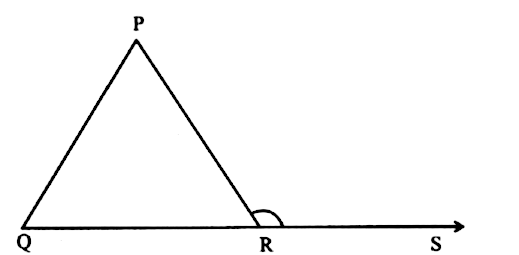Home/Class 7/Maths/

QuestionMathsClass 7

In the given figure, $$\angle PRS=\angle QPR+\angle$$___$$PQR$$
4.64.6Solution

The given figure is:We know that in $$\Delta PQR$$, $$\angle PRS$$ is an exterior angle.
Also, an exterior angle of a triangle is equal to the sum of the two opposite interior angle.
$$\implies \angle PRS=\angle QPR+\angle PQR$$